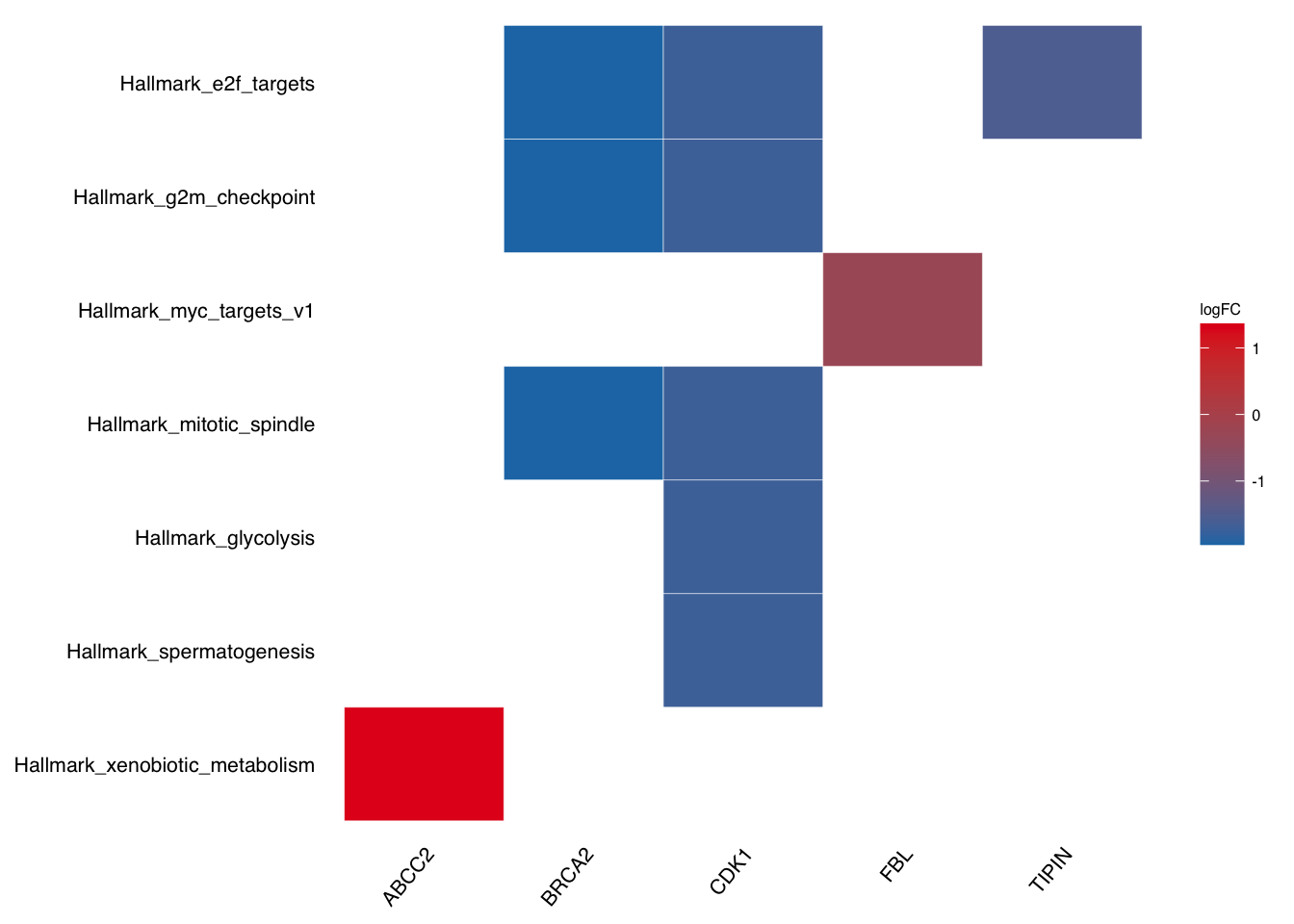# 12 Plot FCS-GSEA

GSEA analysis returns a list result, there are two ways of visulization:

• Directly pass the list to `plotGSEA` which enables 5 types (classic pathway plot, volcano plot, multi-pathway plot, ridge plot and two-side bar plot).

• Extract the analysis result (e.g. `gse_list\$gsea_df`) then pass to `plotEnrich`, which enables 8 types (geneheat, genechord, wordcloud, upset, network, gomap, goheat, gotangram).

## 12.1 Get GSEA result

For more details, please refer to chapter9

``````# 1st step: prepare pre-ranked gene list
data(geneList, package = "genekitr")
``````##       948      1638    158471     10610      6947 100133941
##  5.780170  5.633027  4.683610  3.875120  3.357670  3.322533``````
``````# 2nd step: prepare gene set
gs <- geneset::getMsigdb(org = "human",category = 'H')

# 3rd step: GSEA analysis
gse <- genGSEA(genelist = geneList, geneset = gs)``````

## 12.2 Classic Pathway Plot

Running enrichment score of a gene set is drawn as classic pathway plot lines. The function will walks down the ranked gene list. The vertical lines in the middle show the location of gene set members.

If user wants to show specific pathways or genes, just pass to according parameters: - `show_pathway`: IDs in GSEA result - `show_gene`: IDs in GSEA result

``````pathways <- c("HALLMARK_P53_PATHWAY", "HALLMARK_GLYCOLYSIS", "HALLMARK_DNA_REPAIR")
genes <- c("MET", "TP53", "PMM2")
plotGSEA(gse, plot_type = "classic", show_pathway = pathways, show_gene = genes)``````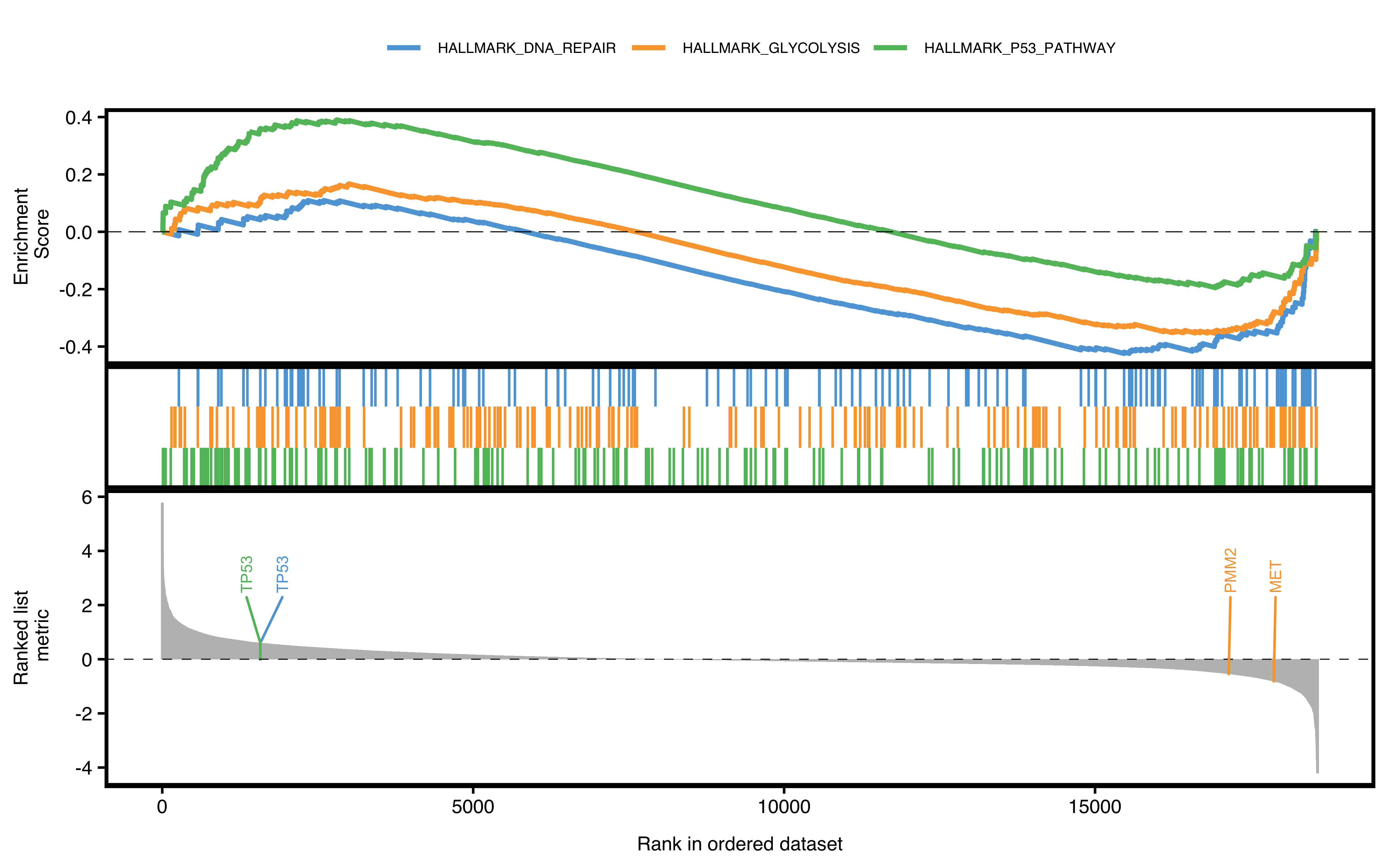Figure 12.1: Classic pathway plot of GSEA.

## 12.3 Volcano Pathway Plot

In this plot, the degree of enrichment is indicated by a normalized enrichment score or NES.

Slightly different with DEG volcano plot, the x-axis is represented as NES, which corrects for enrichment score differences among gene-sets.

A significant positive NES value indicates that members of the gene set tend to appear at the top of the ranked data (e.g. fold change of DEG) and a significant negative NES indicates the opposite.

Default shows the top(N) pathways. If user wants to show specific pathway, also pass the argument to `show_pathway`.

``````library(patchwork)
pathways <- c("HALLMARK_P53_PATHWAY", "HALLMARK_GLYCOLYSIS", "HALLMARK_DNA_REPAIR")
p1 <- plotGSEA(gse, plot_type = "volcano", show_pathway = 3)
p2 <- plotGSEA(gse, plot_type = "volcano", show_pathway = pathways)
p1 + p2 + plot_annotation(tag_levels = "A")``````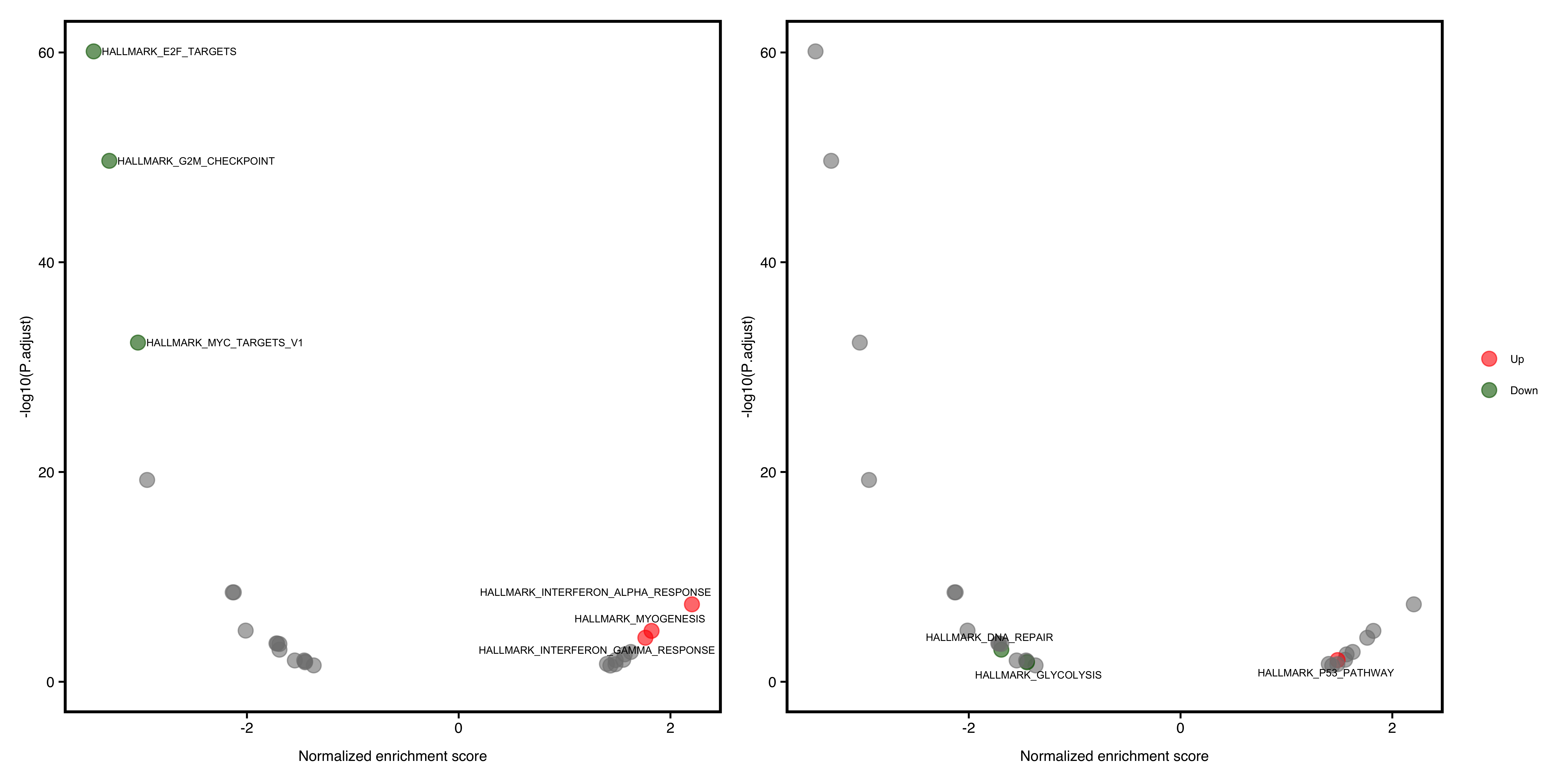Figure 12.2: Volcano pathway plot of GSEA. default (A), select pathways (B).

## 12.4 Multi-pathway Plot

If user wants to show a bunch of selected pathways, `plotGSEA` has scratched some codes from fgsea.

Default shows the top(N) pathways. If user wants to show specific pathway, also pass the argument to `show_pathway`.

``````library(patchwork)
pathways <- c("HALLMARK_P53_PATHWAY", "HALLMARK_GLYCOLYSIS", "HALLMARK_DNA_REPAIR")
p1 <- plotGSEA(gse, plot_type = "fgsea", show_pathway = 5)
p2 <- plotGSEA(gse, plot_type = "fgsea", show_pathway = pathways)
p1 + p2 + plot_annotation(tag_levels = "A")``````Figure 12.3: Multi-pathway plot of GSEA. default (A), select pathways (B).

## 12.5 Ridge Plot

Ridge plot will visualize enriched pathways of up/down regulated genes. The x-axis is fold change and y-axis is pathways. Color represents statistical value (e.g. pvalue/p.adjust/qvalue)

``````plotGSEA(gse,
plot_type = "ridge",
show_pathway = 10, stats_metric = "p.adjust"
)``````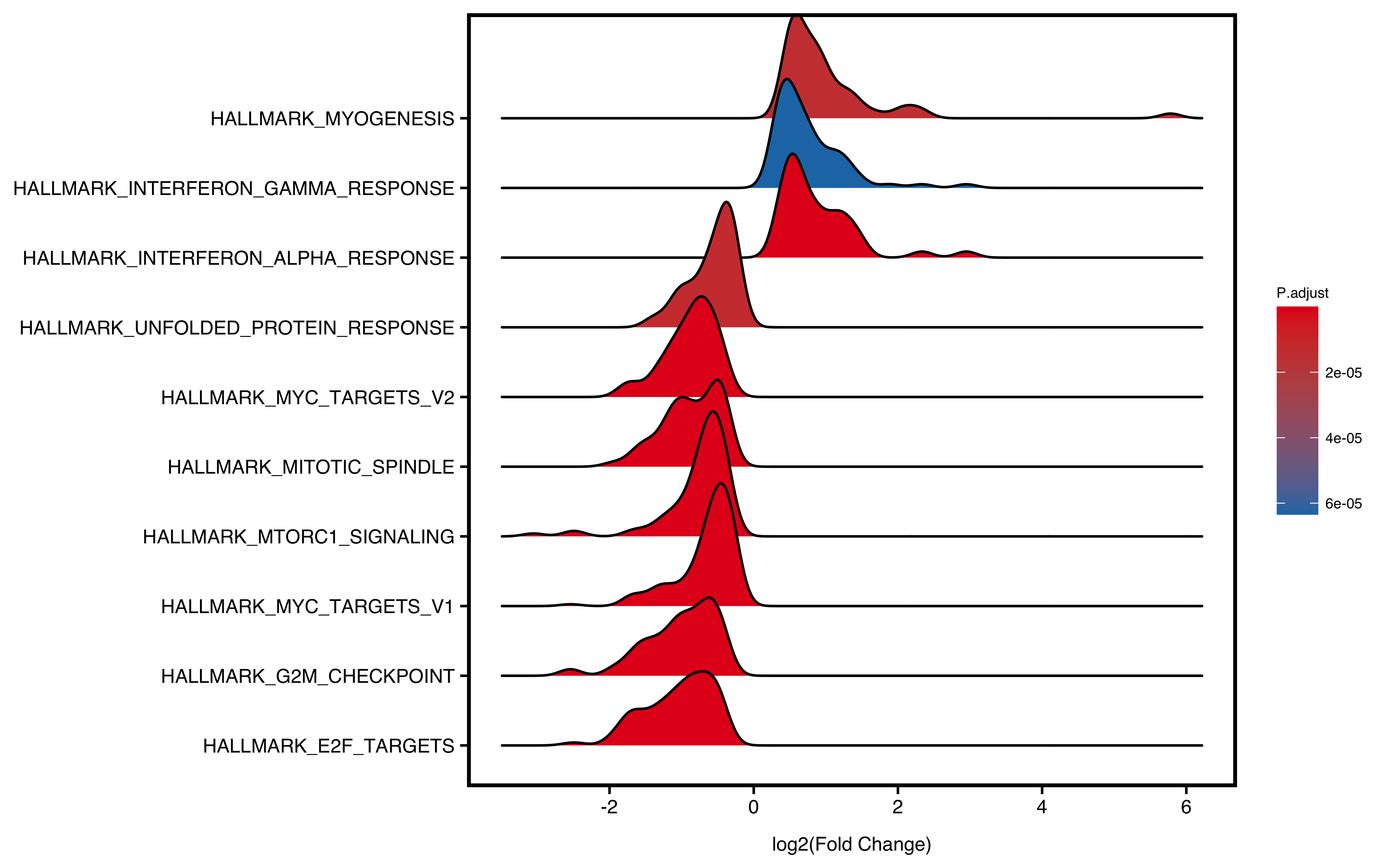Figure 12.4: Ridge plot of GSEA.

## 12.6 Two-side Barplot

The barplot will separate positive and negative NES into two sides. User could specify `color` for the two sides. Meanwhile, non-significant pathways (e.g. Pvalue > 0.05) will be colored as “grey”.

``plotGSEA(gse, plot_type = "bar", colour = c("navyblue", "orange"))``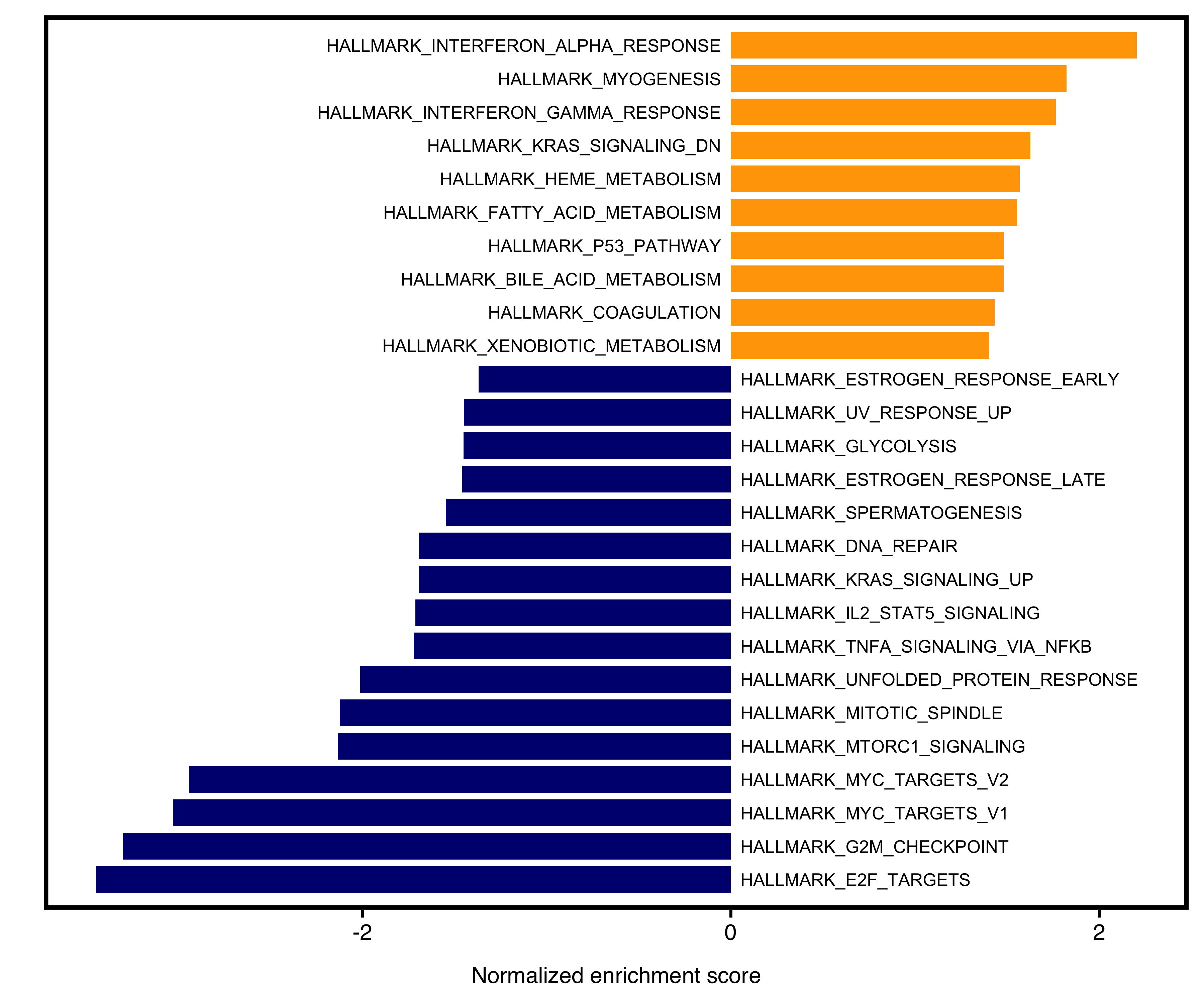Figure 12.5: Two-side bar plot of GSEA.

## 12.7 Borrow from plotEnrich

More plotting details at chapter 11

Take `geneheat` plot as example:

``````plotEnrich(gse\$gsea_df,
plot_type = "geneheat",
show_gene = c("BRCA2", "CDK1", "MCM8", "TIPIN","FBL","ABCC2"),
fold_change = geneList)``````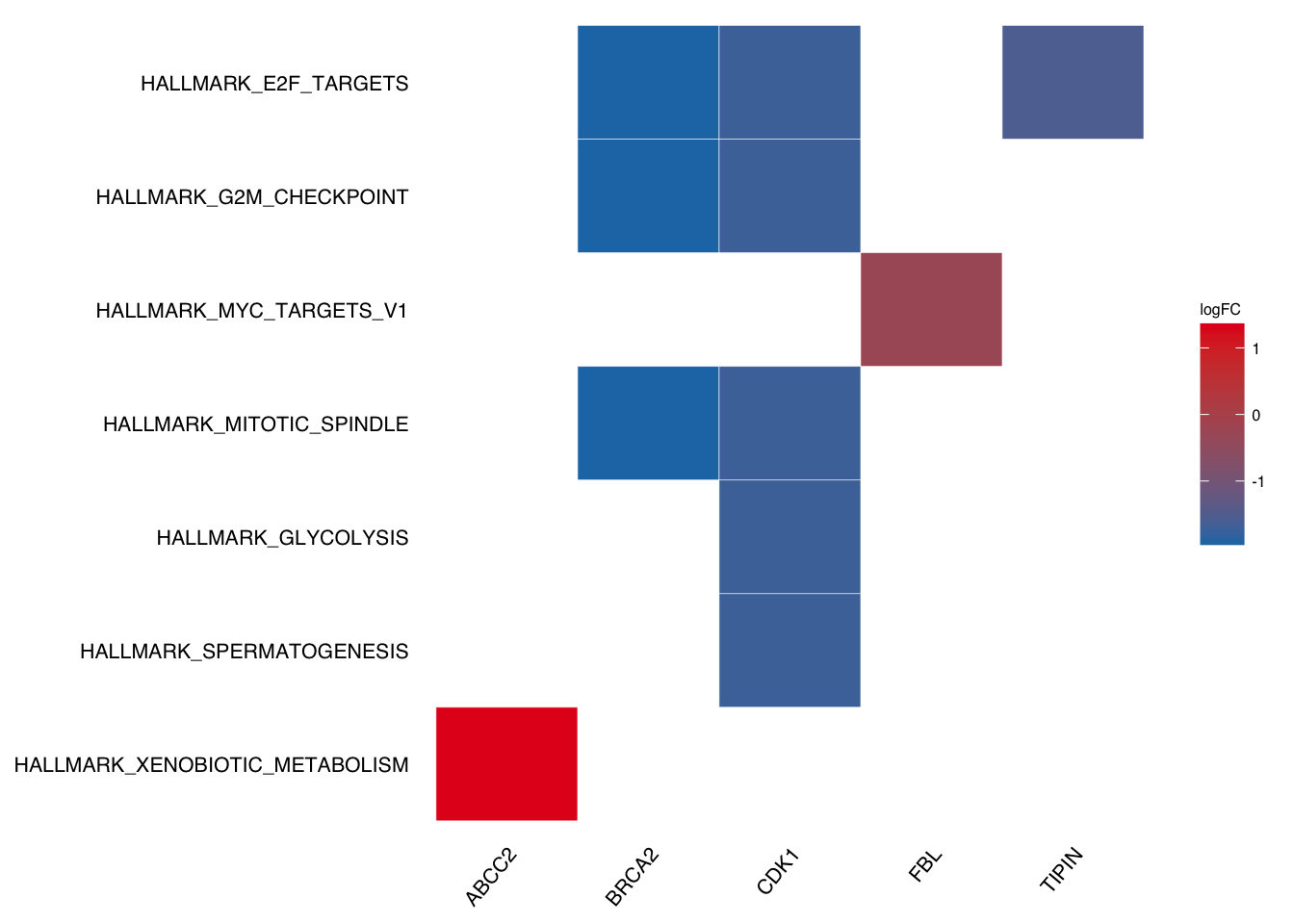## 12.8 Tricks

### 12.8.1 Change figure labels

If we want to DIY labels (e.g. make lower case for above plot), we need to figure out which column the labels from.

In the above `geneheat` plot, the labels are from `Description` column so we only need to change the column.

``````gse2 <- gse
gse2\$gsea_df\$Description <- tolower(gse2\$gsea_df\$Description)
# plotEnrich will uppercase the first letter automatically.
plotEnrich(gse2\$gsea_df,
plot_type = "geneheat",
show_gene = c("BRCA2", "CDK1", "MCM8", "TIPIN","FBL","ABCC2"),
fold_change = geneList)``````